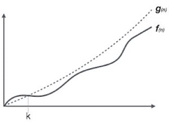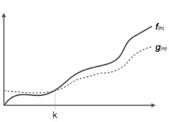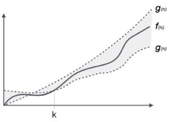Goseeko blog# What is Asymptotic Notation?

Asymptotic notation depicts algorithm efficiency and performance in a meaningful way. It describes how huge instance features behave in terms of time or space complexity.

similarly, The order in which an algorithm’s running time increases gives the algorithm a simple character and allows us to compare the relative performance of different algorithms. Because we ignore the relatively small constant, we term it a growth function. An algorithm’s asymptotic running time is expressed in terms of functions.

When we examine any algorithm, we usually get a formula to represent the amount of time required for execution, or the time required by the computer to run the algorithm’s lines of code, the number of memory accesses, the number of comparisons, the number of temporary variables occupying memory space, and so on. This formula frequently includes unimportant details that provide no information regarding the running time.

## Category  of Asymptotic Notation

The asymptotic notations used to compute the algorithm’s running time complexity are as follows:

1. Big Oh (O) notation
2. Big Omega (ῼ) notation
3. Theta (Ꙫ) notation

## Big Oh (O) Notation

firstly, It is a formal means of expressing the complexity of time. It’s used to set the upper bound on how long an algorithm will take to run. further, It displays the algorithm’s worst-case time complexity, or the amount of time it takes to complete a task.Here, f( n ) = k , C>0 and k>=1

if any algorithm satisfies the eq1 condition then it becomes f ( n )= O  ( g ( n ) )

## Big Omega (ῼ) Notation

It’s used to determine the algorithm’s execution time’s lower bound. In addition, It displays the algorithm’s best case time complexity, or the amount of time it takes to complete a task.Here, f ( n ) >= g ( n )

where n>=k and c>0, k>=1

if any algorithm satisfies the eq1 condition then it becomes f ( n )= ῼ ( g ( n ) )

## Theta (Θ) Notation

It is the formal way to express the time complexity. Additionally, It is used to define the lower as well as upper bound of an algorithm’s running time. Moreover, It displays the average case time complexity, or the amount of time it takes the algorithm to complete a task.Let C1 g(n) be upper  bound and C2 g(n) be lower bound.

C1 g(n)=k

Interested in learning about similar topics? Here are a few hand-picked blogs for you!